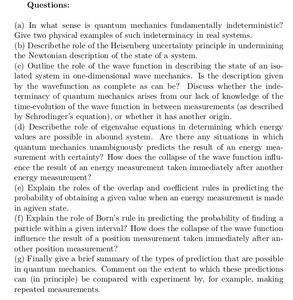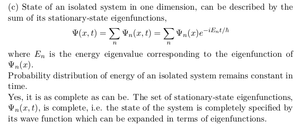Solved Problems in Physics, Physical Chemistry, and Computer Simulation Projects for College and Graduate Students.# Quantum Mechanics: Fundamental Principles

Regular price
\$3.60
Sale price
\$3.60
Tax included.

Questions:

(a) In what sense is quantum mechanics fundamentally indeterministic? Give two physical examples of such indeterminacy in real systems.

(b) Describe the role of the Heisenberg uncertainty principle in undermining the Newtonian description of the state of a system.
(c) Outline the role of the wave function in describing the state of an isolated system in one-dimensional wave mechanics. Is the description given by the wavefunction as complete as can be? Discuss whether the indeterminacy of quantum mechanics arises from our lack of knowledge of the time-evolution of the wave function in between measurements (as described by Schrodinger’s equation), or whether it has another origin.
(d) Describe the role of eigenvalue equations in determining which energy values are possible in a bound system. Are there any situations in which quantum mechanics unambiguously predicts the result of an energy measurement with certainty? How does the collapse of the wave function influence the result of an energy measurement taken immediately after another energy measurement?
(e) Explain the roles of the overlap and coefficient rules in predicting the probability of obtaining a given value when an energy measurement is made in a given state.
(f) Explain the role of Born’s rule in predicting the probability of finding a particle within a given interval? How does the collapse of the wave function influence the result of a position measurement taken immediately after another position measurement?
(g) Finally give a brief summary of the types of prediction that are possible in quantum mechanics. Comment on the extent to which these predictions can (in principle) be compared with experiment by, for example, making repeated measurements.

By purchasing this product, you will get the step by step solution of the above questions in pdf format and the corresponding latex file where you can edit the solution. I am also available to help  you with any possible question you may have.